## If y = 𝑒 𝑥 𝑠𝑖𝑛 2 𝑥 + (sin 𝑥) 𝑥 , find dy/dx .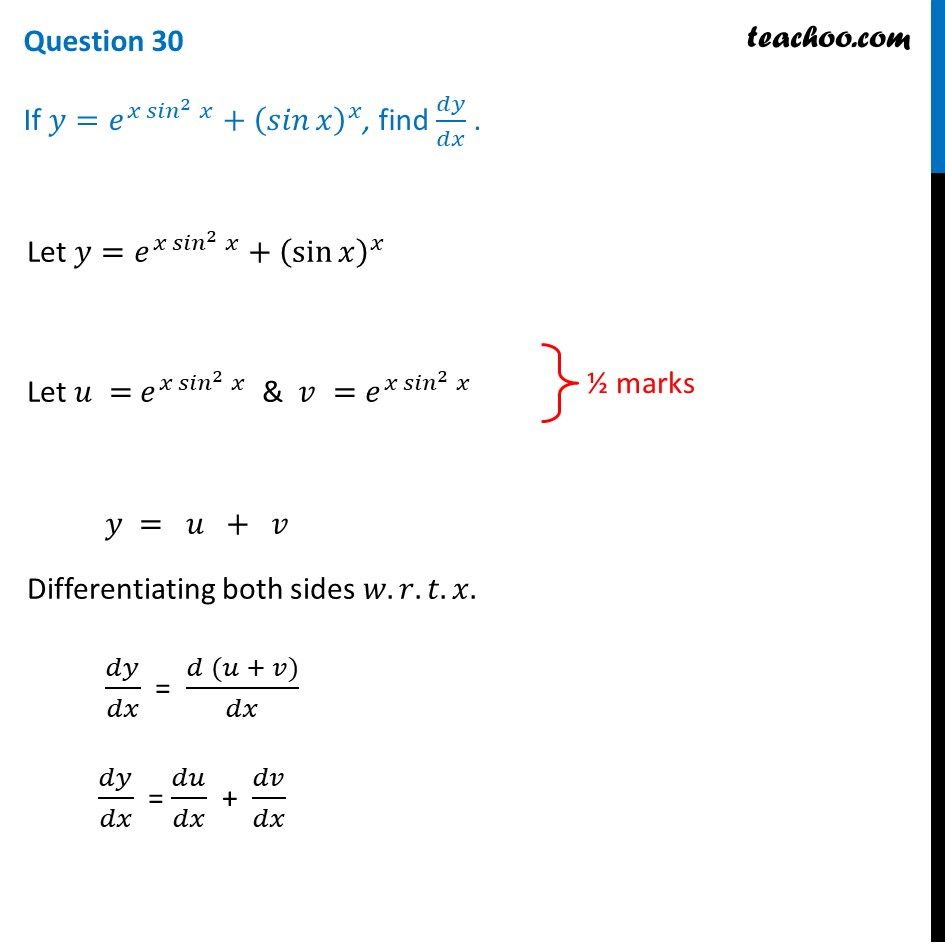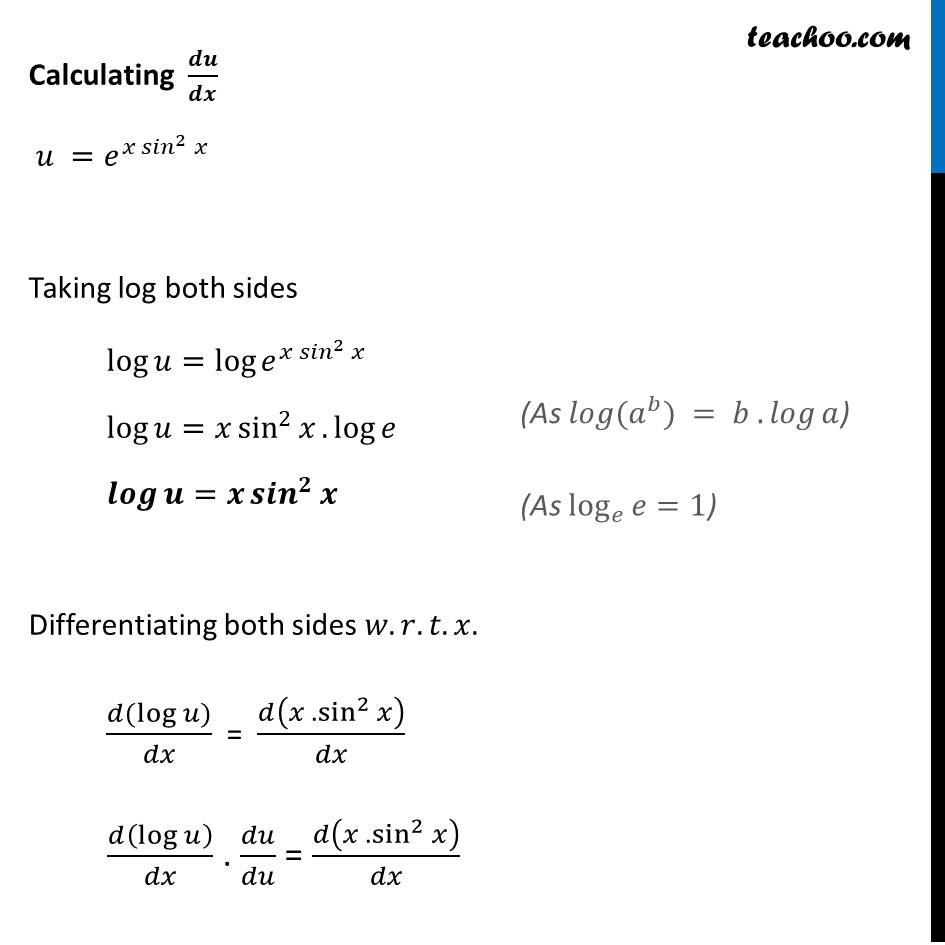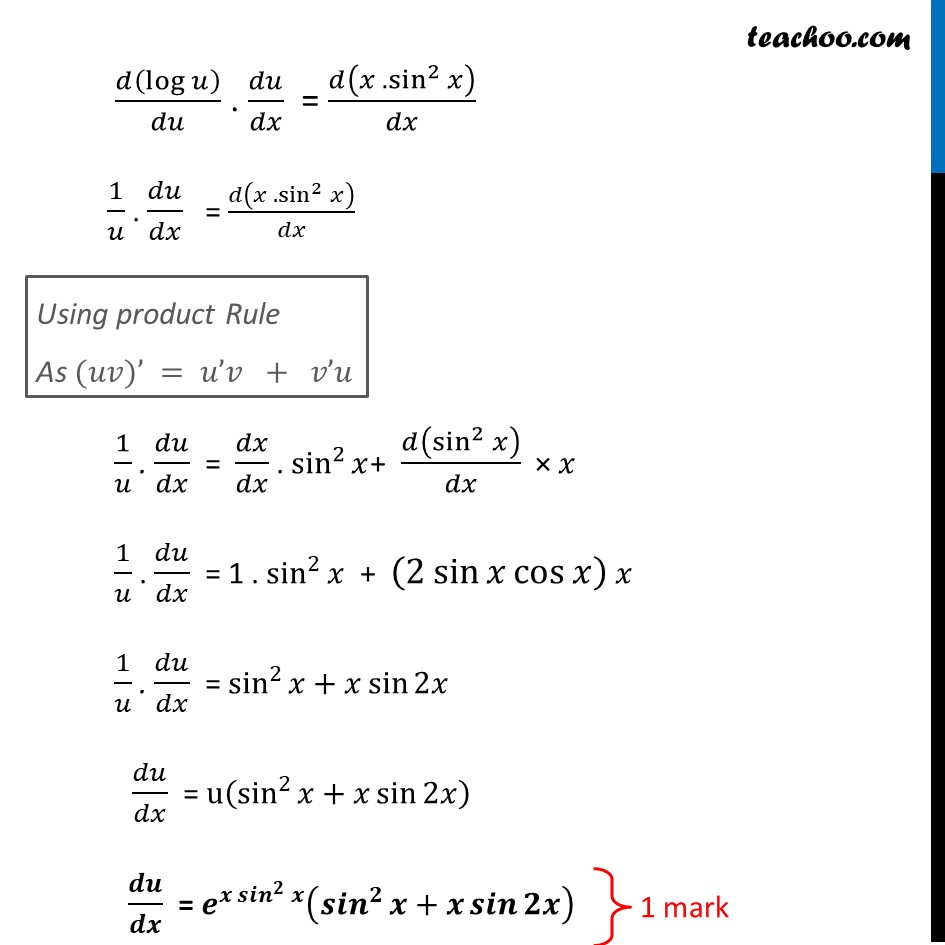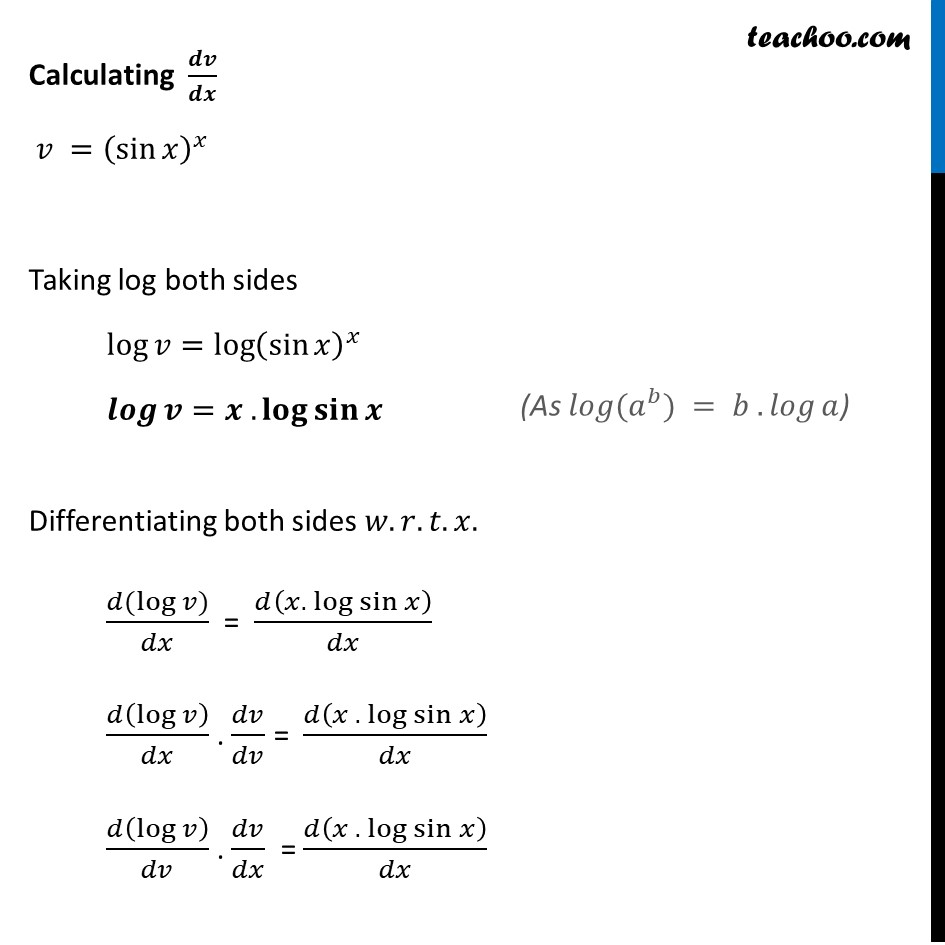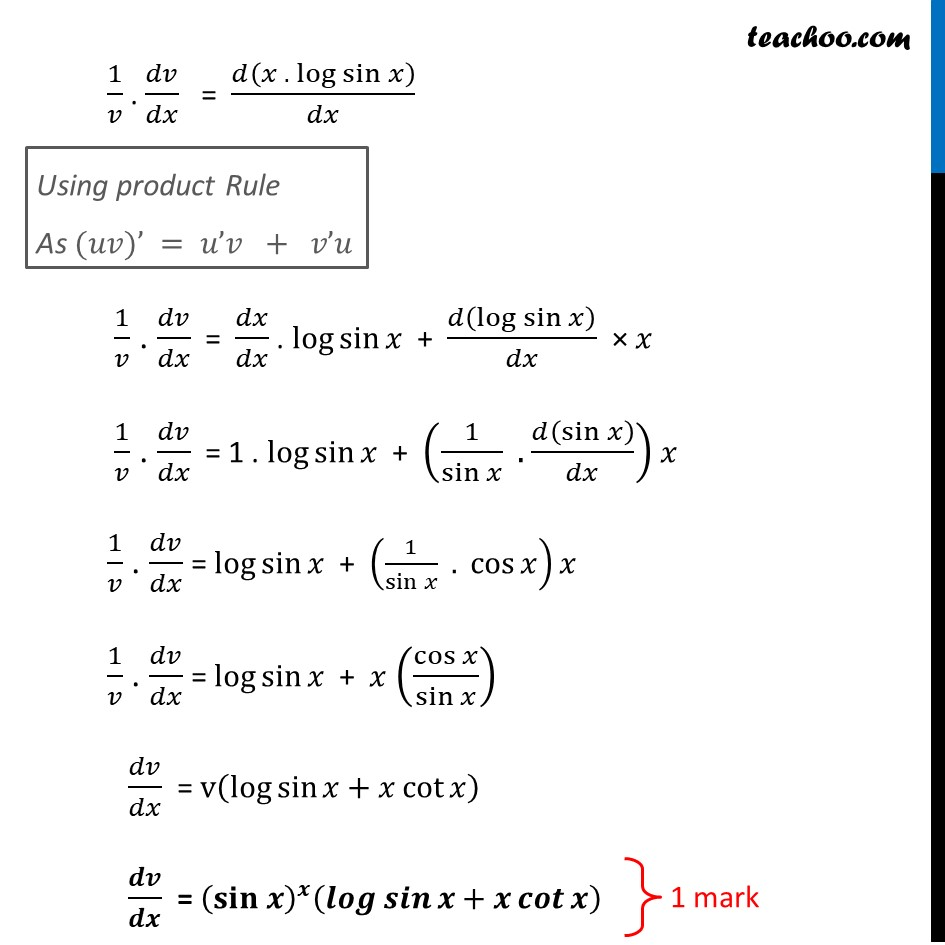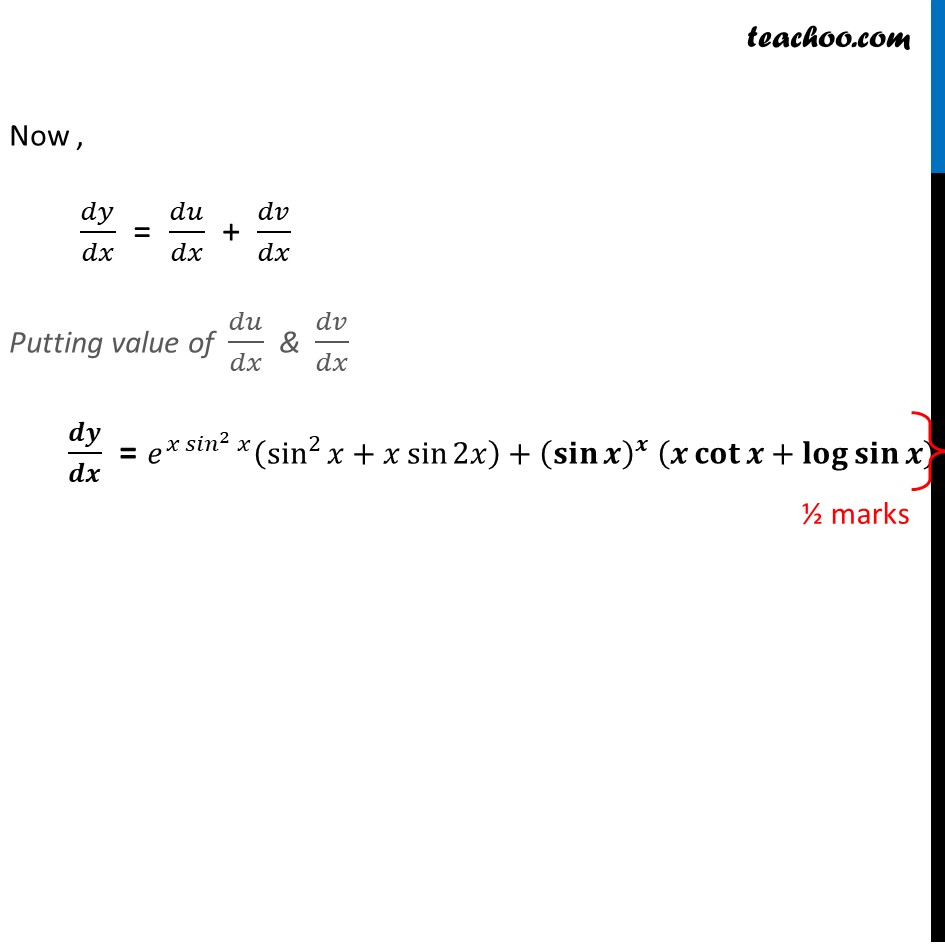1. Class 12
2. Solutions of Sample Papers and Past Year Papers - for Class 12 Boards
3. CBSE Class 12 Sample Paper for 2021 Boards

Transcript

Question 30 If 𝑦=𝑒^(𝑥 〖𝑠𝑖𝑛〗^2⁡𝑥 )+(𝑠𝑖𝑛⁡𝑥 )^𝑥, find 𝑑𝑦/𝑑𝑥 . Let 𝑦=𝑒^(𝑥 〖𝑠𝑖𝑛〗^2⁡𝑥 )+(sin⁡𝑥 )^𝑥 Let 𝑢 = 𝑒^(𝑥 〖𝑠𝑖𝑛〗^2⁡𝑥 ) & 𝑣 =𝑒^(𝑥 〖𝑠𝑖𝑛〗^2⁡𝑥 ) 𝑦 = 𝑢 + 𝑣 Differentiating both sides 𝑤.𝑟.𝑡.𝑥. 𝑑𝑦/𝑑𝑥 = (𝑑 (𝑢 + 𝑣))/𝑑𝑥 𝑑𝑦/𝑑𝑥 = 𝑑𝑢/𝑑𝑥 + 𝑑𝑣/𝑑𝑥 Calculating 𝒅𝒖/𝒅𝒙 𝑢 =𝑒^(𝑥 〖𝑠𝑖𝑛〗^2⁡𝑥 ) Taking log both sides log⁡𝑢=log⁡〖𝑒^(𝑥 〖𝑠𝑖𝑛〗^2⁡𝑥 ) 〗 log⁡𝑢=𝑥 sin^2⁡𝑥.log⁡𝑒 𝒍𝒐𝒈⁡𝒖=𝒙 〖𝒔𝒊𝒏〗^𝟐⁡𝒙 Differentiating both sides 𝑤.𝑟.𝑡.𝑥. (𝑑(log⁡𝑢))/𝑑𝑥 = 𝑑(𝑥 . sin^2⁡𝑥 )/𝑑𝑥 𝑑(log⁡𝑢 )/𝑑𝑥 . 𝑑𝑢/𝑑𝑢 = 𝑑(𝑥 . sin^2⁡𝑥 )/𝑑𝑥 𝑑(log⁡𝑢 )/𝑑𝑢 . 𝑑𝑢/𝑑𝑥 = 𝑑(𝑥 . sin^2⁡𝑥 )/𝑑𝑥 1/𝑢 . 𝑑𝑢/𝑑𝑥 = 𝑑(𝑥 . sin^2⁡𝑥 )/𝑑𝑥 Using product Rule As (𝑢𝑣)’ = 𝑢’𝑣 + 𝑣’𝑢 1/𝑢 . 𝑑𝑢/𝑑𝑥 = 𝑑𝑥/𝑑𝑥 . sin^2⁡𝑥+ 𝑑(sin^2⁡𝑥 )/𝑑𝑥 × 𝑥 1/𝑢 . 𝑑𝑢/𝑑𝑥 = 1 . sin^2⁡𝑥 + (2 sin⁡𝑥 cos⁡𝑥 ) 𝑥 1/𝑢 . 𝑑𝑢/𝑑𝑥 = sin^2⁡𝑥+𝑥 sin⁡2𝑥 𝑑𝑢/𝑑𝑥 = u(sin^2⁡𝑥+𝑥 sin⁡2𝑥 ) 𝒅𝒖/𝒅𝒙 = 𝒆^(𝒙 〖𝒔𝒊𝒏〗^𝟐⁡𝒙 ) (〖𝒔𝒊𝒏〗^𝟐⁡𝒙+𝒙 𝒔𝒊𝒏⁡𝟐𝒙 ) Calculating 𝒅𝒗/𝒅𝒙 𝑣 =(sin⁡𝑥 )^𝑥 Taking log both sides log⁡𝑣=log⁡〖(sin⁡𝑥 )^𝑥 〗 𝒍𝒐𝒈⁡𝒗=𝒙 .𝐥𝐨𝐠⁡𝐬𝐢𝐧⁡〖𝒙 〗 Differentiating both sides 𝑤.𝑟.𝑡.𝑥. (𝑑(log⁡𝑣))/𝑑𝑥 = 𝑑(𝑥.〖 log〗⁡sin⁡𝑥 )/𝑑𝑥 𝑑(log⁡𝑣 )/𝑑𝑥 . 𝑑𝑣/𝑑𝑣 = 𝑑(𝑥 .〖 log〗⁡sin⁡𝑥 )/𝑑𝑥 𝑑(log⁡𝑣 )/𝑑𝑣 . 𝑑𝑣/𝑑𝑥 = 𝑑(𝑥 .〖 log〗⁡sin⁡𝑥 )/𝑑𝑥 1/𝑣 . 𝑑𝑣/𝑑𝑥 = 𝑑(𝑥 .〖 log〗⁡sin⁡𝑥 )/𝑑𝑥 Using product Rule As (𝑢𝑣)’ = 𝑢’𝑣 + 𝑣’𝑢 1/𝑣 . 𝑑𝑣/𝑑𝑥 = 𝑑𝑥/𝑑𝑥 . log⁡sin⁡𝑥 + 𝑑(log⁡sin⁡𝑥 )/𝑑𝑥 × 𝑥 1/𝑣 . 𝑑𝑣/𝑑𝑥 = 1 . log⁡sin⁡𝑥 + (1/sin⁡𝑥 . 𝑑(sin⁡𝑥 )/𝑑𝑥) 𝑥 1/𝑣 . 𝑑𝑣/𝑑𝑥 = log⁡sin⁡𝑥 + (1/sin⁡𝑥 . cos⁡𝑥 )𝑥 1/𝑣 . 𝑑𝑣/𝑑𝑥 = log⁡sin⁡𝑥 + 𝑥 (cos⁡𝑥/sin⁡𝑥 ) 𝑑𝑣/𝑑𝑥 = v(log⁡sin⁡〖𝑥+𝑥 cot⁡𝑥 〗 ) 𝒅𝒗/𝒅𝒙 = (𝐬𝐢𝐧 𝒙)^𝒙 (𝒍𝒐𝒈⁡𝒔𝒊𝒏⁡〖𝒙+𝒙 𝒄𝒐𝒕⁡𝒙 〗 ) Now , 𝑑𝑦/𝑑𝑥 = 𝑑𝑢/𝑑𝑥 + 𝑑𝑣/𝑑𝑥 Putting value of 𝑑𝑢/𝑑𝑥 & 𝑑𝑣/𝑑𝑥 𝒅𝒚/𝒅𝒙 = 𝑒^(𝑥 〖𝑠𝑖𝑛〗^2⁡𝑥 ) (sin^2⁡𝑥+𝑥 sin⁡2𝑥 )+(𝐬𝐢𝐧⁡𝒙 )^𝒙 (𝒙 𝐜𝐨𝐭⁡𝒙+𝐥𝐨𝐠⁡𝐬𝐢𝐧⁡𝒙 )

CBSE Class 12 Sample Paper for 2021 Boards

Class 12
Solutions of Sample Papers and Past Year Papers - for Class 12 Boards